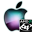ATOMS : Number details

# Number

Integers algorithms
Details
Version
1.2.1
A more recent valid version with binaries for Scilab 5.4 exists: 1.3
Author
Michael Baudin
Owner Organization
Consortium Scilab - DIGITEO
Maintainer
Michael BAUDIN
Category
Dependencies
Creation Date
November 24, 2011
Source created on
Scilab 5.4.x
Binaries available on
Scilab 5.4.x:Windows 64-bitWindows 32-bitLinux 64-bitLinux 32-bitmacOS
Install command
`--> atomsInstall("number")`
Report a bugDescription
```            Purpose
-------

The goal of this toolbox is to provide several Number theory related
algorithms.
The toolbox is based on macros.
The implementation uses Scilab's double precision integers,
therefore is limited to integers in the [-2^53,2^53] interval.

Features
--------

* number_carmichael — Returns some Carmichael numbers.
* number_coprime — Checks if two numbers are relatively prime.
* number_extendedeuclid — Solves a linear Diophantine equation (Bezout
identity).
* number_factor — Factors a number.
* number_fermat — Returns the Fermat number 2^2^n + 1
* number_gcd — Computes the greatest common divisor.
* number_inversemod — Computes the modular multiplicative inverse.
* number_isdivisor — Checks if a divides b.
* number_isprime — Checks if a number is prime.
* number_lcm — Least common multiple.
* number_mersenne — Returns the Mersenne number 2^n - 1
* number_minimumprimegap — Returns the number minimum prime gap.
* number_multiprimality — Returns the number of prime factors of n.
* number_powermod — Modular exponentiation.
* number_primecount — Returns the value of the prime-counting function.
* number_primes — Computes the list of all primes up to n.
* number_primorial — Returns the product of all primes lower or equal to n
* number_probableprime — Check if a number is a probable prime.
* number_pseudoprime — Check if a number is a pseudo prime.
* number_solvelinmod — Solves a linear modular equation.
* number_ulamspiral — Returns the Ulam spiral.

Conversion

* number_barygui — Plots a gui to see the digits of a number.
* number_baryguiclose — Closes the bary GUI.
* number_bin2hex — Converts a binary string into a hexadecimal string.
* number_frombary — Compute a number from a matrix of digits.
* number_hex2bin — Converts an hexadecimal string into a binary string.
* number_tobary — Decompose a number into arbitrary basis.

Support

* number_primes100 — Returns a matrix containing the 100 first primes.
* number_primes1000 — Returns a matrix containing the 1000 first primes.
* number_primes10000 — Returns a matrix containing the 10 000 first primes.
```Files (2)Source code archive
```Same sources as in previous toolbox version, updated to make it available for
Scilab 5.4.0
```OS-independent binary for Scilab 5.4.x
```Binary version
Automatically generated by the ATOMS compilation chain

```News (0)Comments (0)Leave a comment
Login withEmail notifications